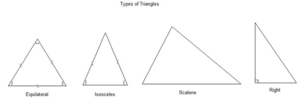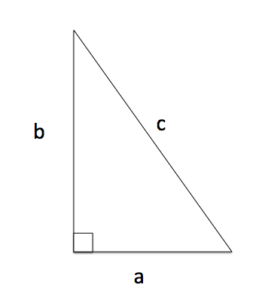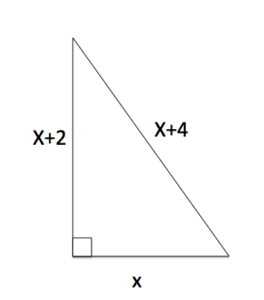## Pythagorean Theorem

### Learning Outcomes

• Solve application problems involving quadratic equations
• Recognize a right triangle from other types of triangles
• Use the Pythagorean theorem to find the lengths of a right triangleTriangles

The Pythagorean theorem, or Pythagoras’s theorem, is a statement about the sides of a right triangle. One of the angles of a right triangle is always equal to $90$ degrees. This angle is the right angle. The two sides next to the right angle are called the legs and the other side is called the hypotenuse. The hypotenuse is the side opposite to the right angle, and it is always the longest side. The image above shows four common kinds of triangle, including a right triangle.Right Triangle with Sides Labeled

The Pythagorean theorem is often used to find unknown lengths of the sides of right triangles. If the longest leg of a right triangle is labeled c, and the other two a, and b as in the image on the left,  The Pythagorean Theorem states that

$a^2+b^2=c^2$

Given enough information, we can solve for an unknown length.  This relationship has been used for many, many years for things such as celestial navigation and early civil engineering projects. We now have digital GPS and survey equipment that have been programmed to do the calculations for us.

In the next example we will combine the power of the Pythagorean theorem and what we know about solving quadratic equations to find unknown lengths of right triangles.

### Example

A right triangle has one leg with length x, another whose length is greater by two,  and the length of the hypotenuse is greater by four.  Find the lengths of the sides of the triangle. Use the image below.This video example shows another way a quadratic equation can be used to find and unknown length of a right triangle.

### Try It

If you are interested in celestial navigation and the mathematics behind it, watch this video for fun.

## Contribute!

Did you have an idea for improving this content? We’d love your input.